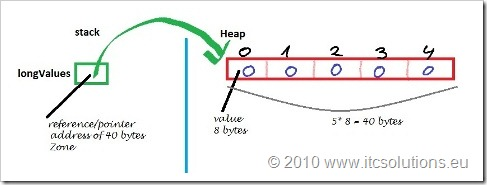# Tutorial Java 6 – #4 Arrays

In this topic we will see how we define and process arrays data structures in Java.

An array is a particular form of Java object that is used to store a homogeneous list of elements (each element of the vector has the same type as vector type – the base type). The number of elements in the array is fixed and it defines its length.

This topic is part of a Java 6 tutorial accessible from Tutorial Java 6 – Contents.

From a syntactically and logically viewpoint, an array in Java is identical to an array in C or C++. There are differences in implementation, but they are transparent to the programmer and you do not notice them:

• in Java, arrays are a special kind of objects – instances of the array class;
• Java allows the use of index operator, [], to access the array elements;
• the new operator (used to build objects and to allocate space in HEAP) accepts as a parameter the array size;

because arrays are objects, the programmer has access to attribute length to get the array size (a major advantage over C or C++ because you have no longer to manage it size by another variable);

#### How we define arrays in Java

The syntax for defining an array in Java:

base_type[] array_name;

base_type array_name[]; // style similar to C/C++

Important! By definition, array_name is an uninitialized variable of type reference (pointer) with a default value equal to null. null is a Java keyword which indicates a nonexistent address with value equal to 0.

```	//defining an array with the recommended syntax for Java
int[] intValues ;
//define array with vector-like sintax in C/C++
long longValues[];```

#### How to initialize arrays in Java

Because they are objects, the array values are stored in HEAP (an area of RAM memory with dynamic allocation in which processes can reserve space at run-time), an array is initialized in 3 steps:

1. Define the array;
2. Reserve space for it;
3. Initialize items value (optional, because during the memory allocation, the elements get default values associated with the base type of the array – for primitive types check and for references the default value is null)

In this tutorial arrays are analyzed with primitive values, and after we see classes and objects related concepts we will analyze arrays of objects.

```         //array definition with Java recommended syntax
int[] intValues;
//define array with vector-like sintax in C/C++
long longValues[];
//allocating space = array initialization
intValues =  new int ; // 10 elements with value 0
longValues =  new long ;   //5 elements with value 0```

Initialization of separate elements is done using operator []. ( WARNING! index used to access the elements has values in [0, length-1])

```        intValues   =  10;    //initialize first element
intValues   =  20;    //initialization of the 2nd element
intValues   =  30;    //initialization of the 3rd element```

If we consider that the array variables (pointers or references) are allocated on main function stack, and the array elements space is reserved in HEAP, then the data memory image is:Memory image of the array and its values

If you know the values of the array, object instantiation and initialization can be done through a single instruction:

base_type[] array_name = {value1, value2, …, valueN);

An example:

```        //allocating space + definition + initialization
//array with five elements with char data type values
char[] charValues = {'H','e','l','l','o'};```

#### How to access and process arrays in Java

Access to array elements is done using operator []. ( WARNING! index used to access the elements has values in the set [0, length-1]).

The number of items in an array is determined by accessing its attribute length (in Java, arrays are objects of type array).

```        char[] charValues = {'H','e','l','l','o'};
//determine the length of the array
int charNumber = charValues.length;
// modify the value of the first element
charValues  =  'H';```

Typically, array processing is done by loop type control structures, for or enhanced for (Java flow control structures are described in another post).

The following example initializes the elements of an array with values from 1 to 10 and then prints them on the console.

```	int[] intValues = new int;

for(int i=0;i < intValues.length;i++)
intValues[i] = i+1;

//enhanced for
for(int value:intValues)
System.out.println(value);```

WARNING! the index used to access the elements takes values in the set [0, length-1]. If the index is not managed properly and gets values that exceeds the array length, then you get runt-time exceptions like ArrayIndexOutOfBoundsException:

```  	char[] charValues = {'H','e','l','l','o'};
// attempt to change the 6th item
// exception at run-time
charValues [ 5 ]  =  '!';```

at run-time you get:

```run:
Exception in thread "main" java.lang.ArrayIndexOutOfBoundsException: 5```

Other common operations with arrays in Java:

Other topics that are part of this Java 6 tutorial are accessible through Tutorial Java 6 – Contents.